# AP SSC 10th Class Maths Notes Chapter 13 Probability

Students can go through AP SSC 10th Class Maths Notes Chapter 13 Probability to understand and remember the concepts easily.

## AP State Syllabus SSC 10th Class Maths Notes Chapter 13 Probability

→ Theory of probability has its origin date back to 16th century.

→ J. Cardan, an Italian physician and mathematician wrote the first book on probability named “The Book of Games of Chance”.→ James Bernoulli (1654 – 1705), A.De Moivre (1667 – 1754) and Pierre Simon Laplace (1749 – 1827) made significant contribution to the theory of probability.

→ Experimental or empirical probability : The probability estimated on the basis of results of an actual experiment is called experimental probability of empirical probability.
Eg : An unbiased coin is tossed for 1000 times, head turned up for 455 times and tail turned up 545 times, then the probability or likelyhood of getting a head is = $$\frac{455}{1000}$$ = 0.455.

Thus experimental probability = $$\frac{\text { No. of trials in which the event happened }}{\text { Total no. of trials }}$$

→ Classical or Theoretical probability: Classical probability of an event (E) is defined Number as

P(E) = $$\frac{\text { Number of outcomes favourable to E }}{\text { No. of all possible outcomes of the experiment }}$$

This definition was given by ‘Pierre Simon Laplace’.
Eg: The probability of getting a head when a coin is tossed is given by Number of outcomes favourable to this event getting a head = 1 Number of all possible outcomes of this experiment = 2 (Head, Tail)

∴ P(E) = $$\frac{\text { No. of favourable outcomes }}{\text { Total events }}$$ = $$\frac{1}{2}$$

Note: If an experiment is conducted for many number of times, then the experimental probability may become closer and closer to theoretical probability.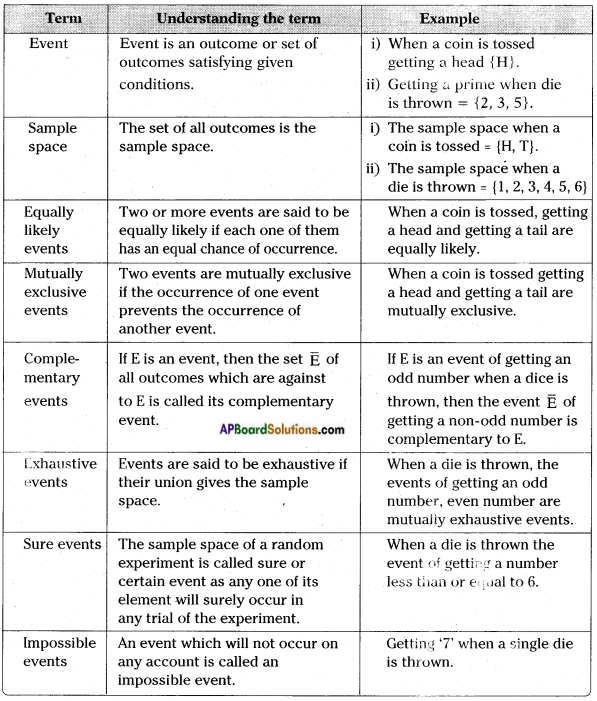→ The probability of a sure event is 1.

→ The probability of an impossible event is zero.

→ The probability of an event E is a number P(E) such that 0 ≤ P(E) ≤ 1.

→ An event having only one outcome is called an elementary event. The sum of the probabilities of all the elementary events of an experiment is 1.

→ For any event E, P(E) + P($$\overline{\mathrm{E}}$$) = 1, where E and $$\overline{\mathrm{E}}$$ are complementary events.

→ Playing cards and their probability : A deck of playing cards consists of 52 cards which are divided into four suits of 13 cards each.
They are: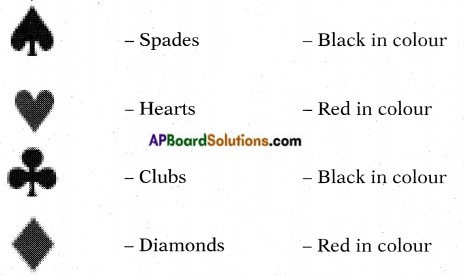→ The cards in each suit are: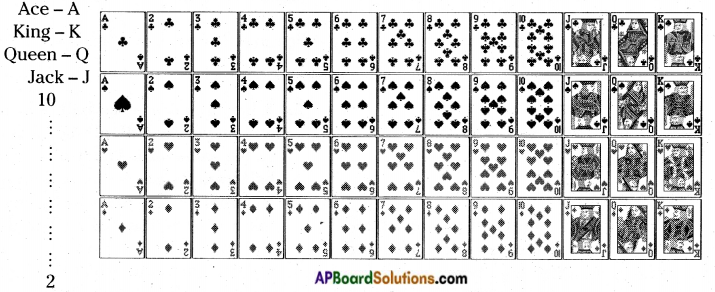Eg : When a card is drawn at random from a deck of cards then

• Getting a black or red card – equally likely exhaustive events.
• Getting an ace or king – mutually exclusive.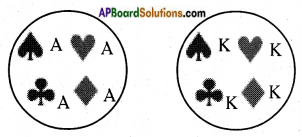• Getting an ace or a hearts – not mutually exclusive since the hearts contain an ace.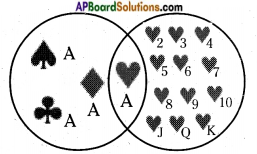→ When a coin is tossed, the outcomes are H, T (Head, Tail).

→ When a dice is thrown the outcomes are 1, 2, 3, 4, 5 and 6.

→ When two dice are thrown, the outcomes are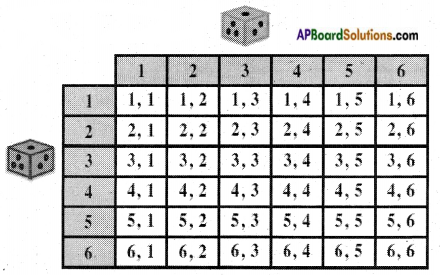→ If a coin is tossed n-times or n – coins are tossed simultaneously, then the number of total outcomes = 2n.

→ If a dice is thrown for n – times or n – dice are thrown simultaneously then the number of total outcomes = 6n.